Courses

# Analog Electronics - 2

## 10 Questions MCQ Test RRB JE for Electronics & Communication Engineering | Analog Electronics - 2

Description
This mock test of Analog Electronics - 2 for Electronics and Communication Engineering (ECE) helps you for every Electronics and Communication Engineering (ECE) entrance exam. This contains 10 Multiple Choice Questions for Electronics and Communication Engineering (ECE) Analog Electronics - 2 (mcq) to study with solutions a complete question bank. The solved questions answers in this Analog Electronics - 2 quiz give you a good mix of easy questions and tough questions. Electronics and Communication Engineering (ECE) students definitely take this Analog Electronics - 2 exercise for a better result in the exam. You can find other Analog Electronics - 2 extra questions, long questions & short questions for Electronics and Communication Engineering (ECE) on EduRev as well by searching above.
QUESTION: 1

### For the transistor circuit shown below: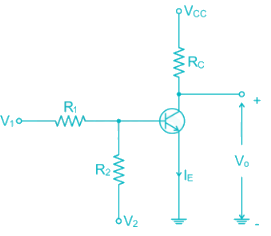If V1 = 1V and V2 = -12V R1 = 15k and R2 = 100k Rc = 2.2 k Vcc = 12V Then the Region of operation of transistor is

Solution:

V1 = 1V

V2 = -12V

R1 = 15 k

R2 = 100 k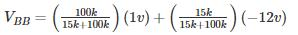= -0.7V

VBB­ < 0, the Base emitter Junction is reversed biased

Hence Transistor is in cut off mode

*Answer can only contain numeric values
QUESTION: 2

### Calculate the value of β for the following circuit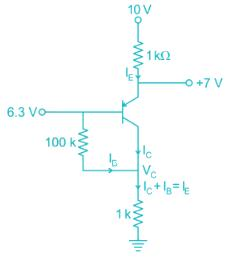Solution: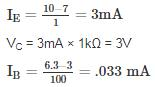I= (1 + β) Iβ

3 × 10-3 = (1 + β) × 0.33 × 10-3

β = 89.9

QUESTION: 3

### In the DC fixed bias circuit shown below the operating point is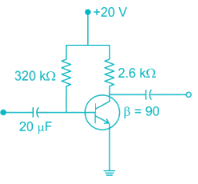Solution: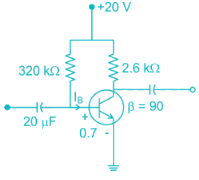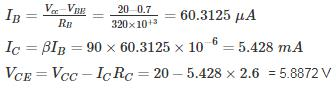So the operating point is (5.43 mA, 5.89 V)

QUESTION: 4

The Miller effect in the context of a Common Emitter amplifier explains

Solution:

The miller effect causes increase in the Input capacitance increase in the Input capacitance of Common – Emitter Amplifier

Upper cutoff frequency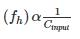Therefore, there is decrease in upper-cut off frequency & Bandwidth of CE Amplifier

*Answer can only contain numeric values
QUESTION: 5

For the network shown in the figure. Find the Input independence Zi (kΩ) [Use appropriate approximation]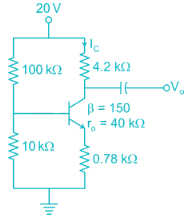Solution:

DC analysis from voltage divider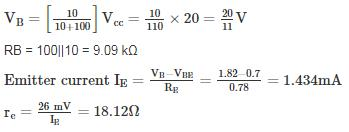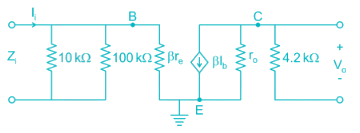Now using appropriate approximations.

Z ≅ βre = 150 × 18.2 = 2.718 kΩ

Input impedance = RB || ZB = 9.09 || 2.718 = 2.092 kΩ

QUESTION: 6

The voltage gain of the amplifier shown if β = 250 is approximately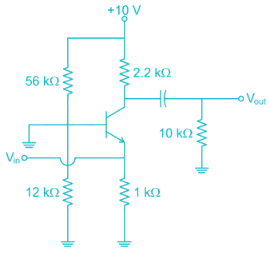Solution:

Form DC Analysis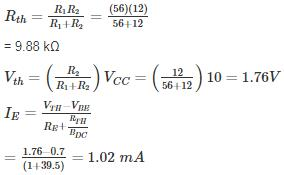Since β value is large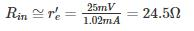Collector resistance = RC’ = RC ||RL

= 2.2 || 10

= 1.8 kΩ

Voltage gain =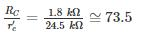*Answer can only contain numeric values
QUESTION: 7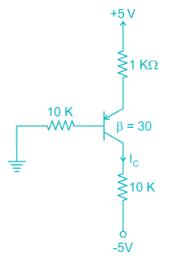For the pnp transistor shown, the collector current IC is_______ mA.

(Assume VEB(ON) = VEB(Sat) = 0.7 V)

VEC(Sat) = 0.2 V

Solution:

Assume transistor is in active region

KVL in base emitter loop

0 + IB (10) + 0.7 + IE (1) = 5

IE = (1 + β)IB

IB (10 + 1 + 30) = 4.3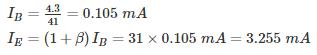IC = βIB = 30 × 0.105 = 3.15 mA

VE = 5 – 1 (3.255)

= 1.745 V

VB = 1.745 – 0.7 = 1.045 V

VC = -5 + 10 (3.15) = 26.5 (not possible)

Transistor is not in active region it is  in saturation region

VEB = 0.7

VE = VB + 0.7

VEC = VB - VC = 0.2

VC = VB + 0.5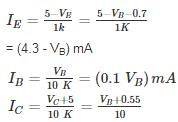= (0.1VB + 0.55) mA

I= IB + IC

4.3 - VB = 0.1 VB + 0.1 VB + 0.55

VB = 3.13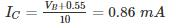QUESTION: 8

For the circuit shown in the figure if RC = 4 kΩ, RL = 4 kΩ, RB = 20 kΩ, Rs = 1 kΩ and transistor parameter are magnitude of hie = 1.1 kΩ, hfe = 50
The current gain is: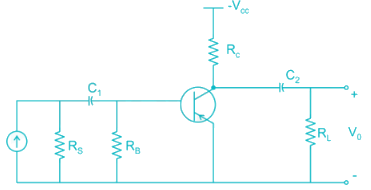Solution:

The AC equivalent circuit is: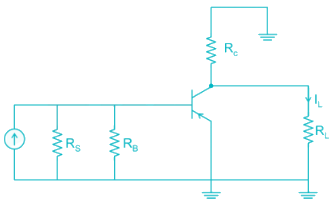Replacing BJT with its h-parameter model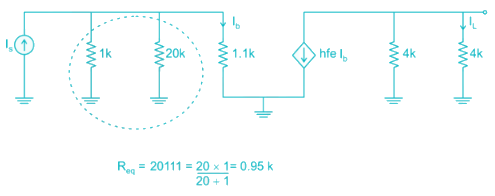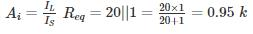ib using current division

iB = 0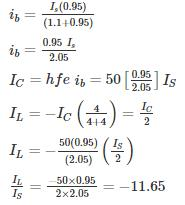Magnitude = |-11.65| ≈ 12

*Answer can only contain numeric values
QUESTION: 9

For the Common emitter configuration circuit shown below, if IC = 1.25 mA, then the magnitude of voltage gain is_______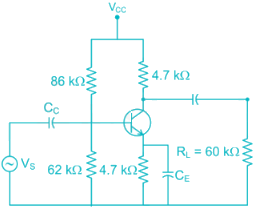Solution:

Given RC = 4.7 kΩ, RL = 60 kΩ, Re = 4.7 kΩ, IC = 1.5 mA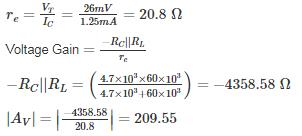QUESTION: 10

The reverse leakage current of the transistor when connected in CB Configuration is 0.3 μ A and it is 18 μA when the same transistor is connected in CE Configuration the value of α and β of the transistor for a base current of 40 mA will be respected.

Solution: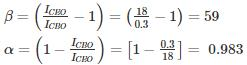Hence α = 0.983 and β = 59